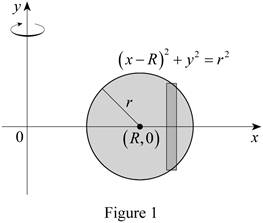# The volume of the solid (torus of Exercise 6.2.63) using cylindrical shell.### Single Variable Calculus: Concepts...

4th Edition
James Stewart
Publisher: Cengage Learning
ISBN: 9781337687805### Single Variable Calculus: Concepts...

4th Edition
James Stewart
Publisher: Cengage Learning
ISBN: 9781337687805

#### Solutions

Chapter 6.3, Problem 36E
To determine

## To find: The volume of the solid (torus of Exercise 6.2.63) using cylindrical shell.

Expert Solution

Thevolume of the solid (torus) is 2π2Rr2_.

### Explanation of Solution

Given:

Solid torus with radii r and R.

The equation of the circle is (xR)2+y2=r2

Show the theorem 7 (Integrals of symmetric Functions):

Apply the condition as follows if the function f is continuous on [–a, a].

1. a) If f is even [f(x)=f(x)], then aaf(x)dx=20af(x)dx.
2. b) If f is odd [f(x)=f(x)], then aaf(x)dx=0.

Calculation:

Show the equation as below:

(xR)2+y2=r2y2=r2(xR)2y=r2(xR)2 (1)

The region lies between x=R+r and x=Rr.

Sketch the solid region as shown in Figure 1.Calculate the volume using the method of cylindricalshell:

V=ab2πx[f(x)]dx (2)

Substitute R+r for a,Rr for b, and 2r2(xR)2 for [f(x)] in Equation (2).

V=RrR+r2πx2r2(xR)2dx=4πRrR+rxr2(xR)2dx (3)

Consider u=xR (4)

Differentiate both sides of the equation.

du=dx

Rearrange Equation (4).

u+R=xx=u+R

Calculate the lower limit value of u using Equation (4).

Substitute R+r for x in Equation (4).

u=R+rR=r

Calculate the upper limit value of u using Equation (4).

Substitute Rr for x in Equation (4).

u=RrR=r

Apply lower and upper limits for u in Equation (3).

Substitute uR for x, u for (rR), and du for dx in Equation (3).

V=4πrr(u+R)r2u2du=4πrr(ur2u2+Rr2u2)du=4πrrur2u2du+4πrrRr2u2du (5)

Consider V1 and V2 as follows:

V1=4πrrur2u2du (6)

V2=4πrrRr2u2du (7)

Substitute V1 for 4πrrur2u2du and V2 for 4πrrRr2u2du in Equation (5).

V=V1+V2 (8)

Consider f(u)=ur2u2 (9)

Substitute (u) for u in Equation (9).

f(u)=ur2(u)2=ur2u2=f(u)

Apply the theorem in Equation (6).

The function satisfies the condition as [f(u)=f(u)].

Thus, the function is an odd function.

Therefore, the volume V1 is 0.

Integrate Equation (7).

V=4πR[12rr2(arcsin(ur)+12sin(2arcsin(ur)))]rr={4πR{[12r2(arcsin(rr)+12sin(2arcsin(rr)))][12r2(arcsin(rr)+12sin(2arcsin(rr)))]}}=4πR{[12r2(π2+12sin(2π2))][12r2(π2+12sin(2π2))]}

=4πR(π4r2+0+π4r2+0)=4πR(π2r2)=2π2Rr2

The volume is,

Substitute 0 for A1 and 2π2Rr2 for A2 in Equation (8)

V=0+2π2Rr2=2π2Rr2

Hence, the volume of the solid (torus) is 2π2Rr2_.

### Have a homework question?

Subscribe to bartleby learn! Ask subject matter experts 30 homework questions each month. Plus, you’ll have access to millions of step-by-step textbook answers!